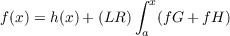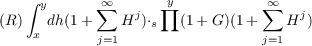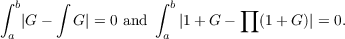#### Vol. 58, No. 1, 1975

 Download this articleFor screen For printingRecent Issues Vol. 325: 1  2 Vol. 324: 1  2 Vol. 323: 1  2 Vol. 322: 1  2 Vol. 321: 1  2 Vol. 320: 1  2 Vol. 319: 1  2 Vol. 318: 1  2Online Archive Volume: Issue:The Journal Subscriptions Editorial Board Officers Contacts Submission Guidelines Submission Form Policies for Authors ISSN: 1945-5844 (e-only) ISSN: 0030-8730 (print) Special Issues Author Index To Appear Other MSP Journals
Product integrals and the solution of integral equations

### Jon Craig Helton

Vol. 58 (1975), No. 1, 87–103
##### Abstract

Functions are from R to N or R × R to N, where R denotes the set of real numbers and N denotes a normed complete ring. If β > 0,H and G are functions from R × R to N,f and h are functions from R to N, each of H,G and dh has bounded variation on [a,b] and |H| < 1 β on [a,b], then the following statements are equivalent:

(1) f is bounded on [a,b], each of abH, abG and (LR) ab(fG + fH) exists andfor a x b, and

(2) each of x y(1 + j=1Hj), x y(1 + G) andexists for a x < y b and

 f(x) = h(a)⋅a ∏ x(1 + G)(1 + ∑ j=1∞Hj) + (R)∫ axdh(1 + ∑ =1∞Hj)⋅ s ∏ x(1 + G)(1 + ∑ j=1∞Hi)
for a x b. This result is obtained without requiring the existence of integrals of the formPrimary: 45D05
##### Milestones